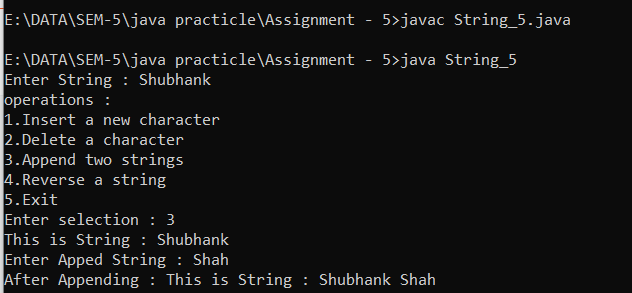# 2 - D Rotation

• A two-dimensional rotation is applied to an object by repositioning it along a circular path in the XY plane.

• To generate a rotation, we specify a rotation angle θ and the position (x, y) of the rotation point (or pivot point) about which the object is to be rotated (Fig. 5-3).

• Positive values for the rotation angle define counterclockwise rotations about the pivot point, as in Fig. 5-3, and negative values rotate objects in the clockwise direction.

• This transformation can also be described as a rotation about a rotation axis that is perpendicular to the XY plane and passes through the pivot point.

• We first determine the transformation equations for rotation of a point position P when the pivot point is at the coordinate origin.

• The angular and coordinate relationships of the original and transformed point positions are shown in Fig. 5-4.

• In this figure, r is the constant distance of the point from the origin, angle

• Φ is the original angular position of the point from the horizontal, and θ is the rotation angle.

• Using standard trigonometric identities, we can express the transformed coordinates in terms of angles θ and Φ as:

Figure (5.4)

x' = r cos (Φ + θ) = r cosΦ cosθ - r sinΦ sinθ

y' = r sin (Φ + θ) = r cosΦ sinθ - r sinΦ cosθ

• The original coordinates of the point in polar coordinates are

Figure (5.5)

x = r cos Φ, y = r sin Φ

• Substituting expressions 5-5 into 5-4, we obtain the transformation equations for rotating a point at position (x, y) through an angle θ about the origin:

Figure (5.6)

x' = xcosθ - ysinθ

y' = xsinθ + y cosθ

• With the column-vector representations 5-2 for coordinate positions, we can write the rotation equations in the matrix form:

Figure (5.7)

P' = R * P

Where the rotation matrix is:

Figure (5.8)

R= [ [cosθ - sinθ] [sinθ cosθ] ]

• When coordinate positions are represented as row vectors instead of column vectors, the matrix product in rotation equation 5-7 is transposed so that the transformed row coordinate vector |x' y'| is calculated as

P't = (R * P) t

= Pt * Rt

• Where PT = [x y], and the transpose RT of matrix R is obtained by interchanging rows and columns.

• For a rotation matrix, the transpose is obtained by simply changing the sign of the sine terms.

• The rotation of a point about an arbitrary pivot position is illustrated in Fig. 5-5.

• Using the trigonometric relationships in this figure, we can generalize Eqs. 5-6 to obtain the transformation equations for rotation of a point about any specified position (x,,!~,):

Figure (5.9)

X ' = X, + (a - x,) cos θ - (y - y,) sin θ

Y' = Y, + (1 - v,) sin θ + (y - y,) cos θ

• These general rotation equations differ from Eqs. 5-6 by the inclusion of additive terms, as well as the multiplicative factors on the coordinate values.

• Thus, the matrix expression 5-7 could be modified to include: pivot coordinates by matrix addition of a column vector whose elements contain the additive (translational) terms In Eqs. 5-9.

• There are better ways, however, to formulate such matrix equations, and we discuss in Section 5-2 a more consistent scheme for representing the transformation equations.

• As with translations, rotations are rigid-body transformations that move objects without deformation.

• Every point on an object is rotated at the same angle.

• A straight line segment is rotated by applying the rotation equations 5-9 to each of those' line endpoints and redrawing the line between the new endpoint positions.

• Polygons are rotated by displacing each vertex through the specified rotation angle and regenerating the polygon using the new vertices.

• Curved lines arc rotated by repositioning the defining p ~ r ~atnsd redrawing the curves.

• A circle k>r,117 ellipses, for instance, can be rotated about a noncentral axis by moving the center position through the arc that substances the specified rotation angle.

• An ellipse can be rotated about its center coordinates by rotating the major and minor axis.

Concept of Char_Up Vector In Computer Graphics

Liang Barsky Algorithm